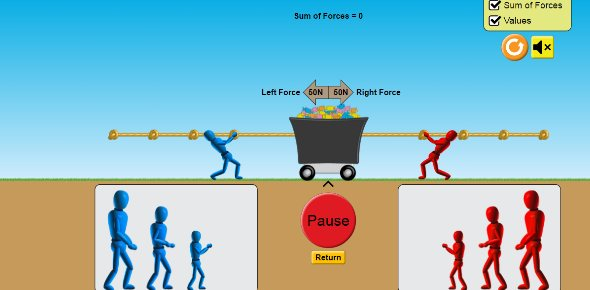# Energy Force And Motion

10 Questions | Attempts: 222
ShareSettings• 1.
What is patricas average speed if she ran 60 kilometers in 6 seconds?
• A.

5

• B.

10

• C.

320

• 2.
What is patricas average speed if she ran 60 kilometers in 6 seconds?
• A.

5

• B.

10

• C.

320

• 3.
True or False.Everthing has some kind of force on them?
• A.

True

• B.

False

• 4.
True or False.Everthing has some kind of force on them?
• A.

True

• B.

False

• 5.
What is the formula for calculating speed?
• A.

Speed=velocity/distance

• B.

Speed=distance/speed

• C.

Speed=speed

• 6.
What is the formula for calculating speed?
• A.

Speed=velocity/distance

• B.

Speed=distance/speed

• C.

Speed=speed

• 7.
True or false.Gravity is the pull that pulls you to the center of the earth?
• A.

True

• B.

False

• 8.
True or false.Gravity is the pull that pulls you to the center of the earth?
• A.

True

• B.

False

• 9.
True or False.There are six basic simple machines?
• A.

True

• B.

False

• 10.
True or False.There are six basic simple machines?
• A.

True

• B.

False

## Related TopicsBack to top
×

Wait!
Here's an interesting quiz for you.